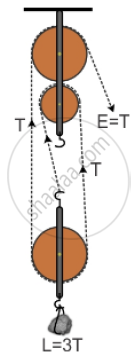Share
Notifications

View all notifications
Books Shortlist
Your shortlist is empty

# A Pulley System Has a Velocity Ratio 3 and an Efficiency of 80%. Draw a Labelled Diagram of this Pulley System Calculate: (A) the Mechanical Advantage of the System and (B) the Effort Required to - ICSE Class 10 - Physics

Login
Create free account

Forgot password?

#### Question

A pulley system has a velocity ratio 3 and an efficiency of 80%. Draw a labelled diagram of this pulley system Calculate:
(a) the mechanical advantage of the system and

(b) the effort required to raise a load of 300 N.

#### SolutionA pulley system has a velocity ratio = 3
Efficiency of system = 80 % = 0.8
Mechanical advantage of the system M.A = V.A × η = 3 × 0.8 = 2.4
Effort required to raise the load = Effort = "𝐿𝑜𝑎𝑑 "/"𝑀.𝐴"= 300/2.4  =  125N

Is there an error in this question or solution?

#### APPEARS IN

Solution A Pulley System Has a Velocity Ratio 3 and an Efficiency of 80%. Draw a Labelled Diagram of this Pulley System Calculate: (A) the Mechanical Advantage of the System and (B) the Effort Required to Concept: Concept of Pulley System.
S